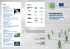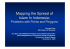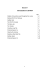# paper [PDF:1.7MB]

## Transcription

paper [PDF:1.7MB]
```TP
RIETI Technical Paper Series 15-T-001
Hot and Cold Spot Analysis Using Stata
KONDO Keisuke
RIETI
The Research Institute of Economy, Trade and Industry
http://www.rieti.go.jp/en/
RIETI Technical Paper Series 15-T-001
October 2015
Hot and Cold Spot Analysis Using Stata*
KONDO Keisuke†
RIETI
Abstract
Spatial analysis is attracting more attention from Stata users with the increasing
availability of regional data. This article presents an implementation of hot and cold
spot analysis using Stata. For this purpose, I introduce the new command getisord,
which calculates the Getis–Ord 𝐺𝑖∗ (𝑑) statistic in Stata. To implement this command,
the only additionally required information is the latitude and longitude of regions. In
combination with shape files, results obtained from the getisord command can be
visually displayed in Stata. In this article, I offer an interesting illustration to explain
how the getisord command works in Stata.
Keywords: getisord, Getis–Ord 𝐺𝑖∗ (𝑑), Local spatial autocorrelation, Shape file
The views expressed in the papers are solely those of the author(s), and neither represent those
of the organization to which the author(s) belong(s) nor the Research Institute of Economy,
This is a research outcome undertaken at the Research Institute of Economy, Trade and Industry.
Research Institute of Economy, Trade and Industry (RIETI): 1-3-1 Kasumigaseki, Chiyoda-ku, Tokyo, 100-8901,
Japan. (E-mail: [email protected])
*
†
Hot and cold spot analysis using Stata
1
2
Introduction
Spatial analysis is becoming more popular with the increasing availability of geographically
disaggregated data as well as map ﬁles (e.g., shape ﬁles), and hence, there is a growing demand
for spatial analysis using Stata among researches worldwide. However, Stata packages that
specialize in spatial analysis are not currently suﬃcient due to some computational diﬃculties.
This article intends to ﬁll this gap by introducing the new command getisord for hot and cold
spot analysis.
Our socioeconomic activities are concentrated in certain locations in the real world, and the
spatial pattern is not randomly distributed. Thus, one of the purposes of spatial analysis is
to describe how our socioeconomic activities are distributed in space. To detect the hot and
cold spots, Getis and Ord (1992) develop the Getis–Ord G∗i (d) statistic, a measure of spatial
autocorrelation from a local perspective.
Spatial autocorrelation is an important concept in literature and comprises two strands.
Global spatial autocorrelation such as Moran’s I looks at the overall spatial interdependence
between regions. On the other hand, local spatial autocorrelation such as Getis–Ord G∗i (d) is
motivated by the idea that the spatial association may be locally heterogeneous even if global
spatial autocorrelation is observed. In this context, Getis and Ord (1992) develop a method for
hot and cold spot analysis in a geographical space. Furthermore, this method is extended to the
generalized case by Ord and Getis (1995) to ﬂexibly consider degrees of spatial connections.1
The getisord command introduced in this article allows us to calculate Getis–Ord G∗i (d)
with binary and non-binary spatial weight matrices. To implement the getisord command,
geographical information on latitude and longitude is required. In other words, researchers can
easily conduct hot and cold spot analysis as long as datasets contain basic regional information,
such as zip code, city code, and city name. The recent geocoding technique facilitates the
addition of geographical information on latitude and longitude into the dataset, even if a suitable
shape ﬁle of the corresponding area is not available.2
Naturally, if a shape ﬁle is available, the getisord command becomes a more powerful
tool. Visualization helps us foster better understanding of the spatial analysis. Fortunately,
Stata already provides the shp2dta command that converts the shape ﬁle to the Stata DTA
ﬁle (Crow, 2015). In addition, results obtained from the getisord command can be visualized
in Stata in combination with the spmap command that displays regional data in map (Pisati,
2008).
This article provides an interesting illustration of the getisord command. Using the US
county data of median family income from 1959 to 1989, the getisord command clariﬁes which
counties in the US have formed high-income clusters from spatial and dynamic perspectives. A
similar analysis is conducted in Kondo (2015), which detects Japanese unemployment clusters
that permanently show high unemployment rates regardless of temporal ﬂuctuations from 1980
1
Getis and Ord (1992) also propose the Getis–Ord Gi (d) statistic that does not include own value. This article
focuses only on Getis–Ord G∗i (d), which is frequently used in empirical analyses.
2
For example, the Google Maps geocoding API is publicly available.
Hot and cold spot analysis using Stata
3
to 2005.
statistics and spatial econometrics. Researchers often face a diﬃculty to deal with spatial
weight matrix in Stata, which makes the spatial analysis diﬃcult within the Stata framework.
An outstanding command for spatial econometrics in Stata is the spreg command oﬀered by
Drukker et al. (2013). However, including a spatial weight matrix into Stata using a shape ﬁle
might be a strict requirement for a part of researchers; a suitable shape ﬁle is not available in
some situations. In turn, the spatial weight matrix in the getisord command is constructed
only from the geographical information on latitude and longitude; it is easily available via the
recent geocoding technique.3 Therefore, this article also contributes to the construction method
of a spatial weight matrix in Stata, which facilitates further development of Stata packages for
spatial analysis.
The rest of this paper is organized as follows. Section 2 reviews the Getis–Ord G∗i (d)
statistic. Section 3 explains how the bilateral distance is measured in Stata. Section 4 describes
the getisord command. Section 5 oﬀers an example, and Section 6 presents the conclusions.
2
Detecting hot and cold spots
Getis and Ord (1992) develop a method for hot and cold spot analysis in a geographical space.
The Getis-Ord G∗i (d) statistic is calculated as follows (Getis and Ord, 1992):
G∗i (d)
N
=
j=1 wij (d)xj
,
N
j=1 xj
(1)
where wij (d) denotes ijth element of the spatial weight matrix as follows:
⎧
⎨1, if d < d,
ij
wij (d) =
⎩0, otherwise.
for all i, j
(2)
Note the diagonal elements take the value of 1 because of dii = 0. Hereafter, I use the notation
wij (d) to denote a general form of spatial weight matrix, including a non-binary spatial weight
matrix.
The essence of Getis–Ord G∗i (d) is as follows. The numerator in equation (1) gives the local
sum of variable x within a circle of d km radius from the base point (e.g., centroid) of region i,
and the denominator in equation (1) gives the total sum of variable x for all the regions. The
Getis–Ord G∗i (d) statistic evaluates the ratio of the local sum to the total sum for each region.
Therefore, hot and cold spots are detected as spatial outliers.
Ord and Getis (1995) extend this statistic to the case of the non-binary spatial weight
3
The key point is that the spatial weight matrix is endogenously constructed in a sequence of the program
code and not exogenously included into Stata as a matrix type. Although this method based on the latitude and
longitude can be easily conducted, the spatial weight matrix is limited to the case of great-circle distance.
Hot and cold spot analysis using Stata
4
matrix. The generalized formula of Getis–Ord G∗i (d) is deﬁned for both the binary and non-
binary spatial weight matrices. The standardized Getis–Ord G∗i (d), which is equivalent to the
z-value of Getis–Ord G∗i (d), is given by
Standardized G∗i (d) =
G∗i (d) − E[G∗i (d)]
.
Var[G∗i (d)]
Under the complete spatial randomness, the expectation and variance of the Getis–Ord G∗i (d)
are, respectively, derived as follows:
E[G∗i (d)]
N
j=1 wij (d)
=
Var[G∗i (d)] =
N
N
N
,
2
j=1 wij (d) −
N
2 2
s
j=1 wij (d)
N 2 (N − 1)
x̄
(3)
,
where x̄ is the sample mean and s2 is the sample variance.4
The distribution of the standardized G∗i (d) approaches a standard normal distribution as
N approaches inﬁnity. When the standardized G∗i (d) takes a positive (negative) value and falls
within the critical region, region i is identiﬁed as a hot (cold) spot. The critical values for
hot and cold spots are approximately ±1.96 and ±2.58 at the 5% and 1% signiﬁcance levels,
respectively. In a similar manner, the p-value is obtained from the cumulative distribution
function of the standard normal distribution.
For the standardized Getis–Ord G∗i (d), several types of non-binary spatial weight matrix
can be considered. For example, the case of power function is shown below:
⎧
⎨(a + d )−δ , if d < d,
ij
ij
wij (d) =
⎩0,
otherwise,
for all i, j,
δ > 0,
(4)
where δ is a distance decay parameter and a is the constant value added to avoid wii = ∞ due
to dii = 0. Ord and Getis (1995) consider the case of inverse distance matrix (δ = 1, d = ∞).
Another case is the exponential type of spatial weight matrix as follows:
⎧
⎨exp(−δd ),
ij
wij (d) =
⎩0,
if dij < d,
for all i, j,
δ > 0,
(5)
otherwise,
where δ is the distance decay parameter. Compared to the power type of the spatial weight
matrix, an advantage of the exponential type is that it is unnecessary to decide constant a
beforehand because the own weight is wii = 1 for dii = 0.
4
The variance in equation (3) cannot be deﬁned if the numerator of the variance takes the value of 0. In this
case, try diﬀerent threshold distance d in the binary spatial weight matrix or diﬀerent distance decay parameter
δ in the non-binary spatial weight matrix.
Hot and cold spot analysis using Stata
3
5
Measuring distance
This article uses the Vincenty formula to measure bilateral distance between regions (Vincenty,
1975). To fasten computational time in the case where the number of regions is too large, the
getisord command oﬀers a simpliﬁed version of the Vincenty formula.
3.1
Vincenty formula
The Vincenty formula is commonly used to measure the geographical distance between two
points on Earth. Unlike the spherical law of cosines and the haversine formula, Vincenty (1975)
proposes a method measuring geodesic distance under the condition where the shape of the
Earth is ellipsoidal.
Given latitudes and longitudes of two locations, the getisord command measures the bilateral distance by using the Vincenty formula, considering that the shape of the Earth is not
a perfect sphere. See Vincenty (1975) for further details of the calculation procedure.
3.2
Simplified version of Vincenty formula
The Vincenty formula contains an iteration procedure to improve the accuracy. However, the
computation of bilateral distance takes considerable time when the number of regions is large.5
To shorten computational time, the getisord command oﬀers the approx option, which measures bilateral distance by using a simpliﬁed version of the Vincenty formula.
The great-circle distance is basically calculated by
dij = r × θ,
where r is the radius of the Earth (≈ 6378.137 km) and θ is the central angle of the arc
between two locations. Given the latitudes and longitudes of two locations, θ is calculated by
the spherical law of cosines or the haversine formula.
Let φi and λi denote the latitude and longitude of region i in radians. Following Vincenty
(1975), θ is calculated from the inverse of tan θ = sin θ/ cos θ as follows:
⎛ θ = arctan ⎝
2 2 ⎞
cos(φj ) sin(Δλ) + cos(φi ) sin(φj ) − sin(φi ) cos(φj ) cos(Δλ)
⎠,
sin(φi ) sin(φj ) + cos(φi ) cos(φj ) cos(Δλ)
where Δλ = λj − λi .
I conﬁrm that this approximation works very well even without iteration of the exact process
in the Vincenty formula. The comparison between the exact and approximated procedures of
the Vincenty formula will be given in an applied example.
5
Since the distance matrix is symmetric, N (N − 1)/2 iterations are needed.
Hot and cold spot analysis using Stata
4
6
Implementation in Stata
4.1
Syntax
getisord varname
if
approx constant(#)
in
, lat(varname) lon(varname) swm(swmtype) dist(#)
dms
The getisord command calculates the Getis–Ord G∗i (d) statistic of varname.
4.2
Options
lat(varname) speciﬁes the variable of latitude in the dataset. The decimal format is expected
in the default setting. The positive value denotes the north latitude. The negative value
denotes the south latitude.
lon(varname) speciﬁes the variable of longitude in the dataset. The decimal format is expected
in the default setting. The positive value denotes the east longitude. The negative value
denotes the west longitude.
swm(swmtype) speciﬁes a type of spatial weight matrix. One of the following three types of
spatial weight matrix must be speciﬁed: bin (binary), exp (exponential), or pow (power).
The distance decay parameter must be speciﬁed for the exponential and power functional
types of spatial weight matrix as follows: swm(exp #) and swm(pow #).
dist(#) speciﬁes the threshold distance for the spatial weight matrix.
dms converts the degrees, minutes and seconds (DMS) format to a decimal. The dms option is
not used in the default setting.
approx uses bilateral distance approximated by the simpliﬁed version of the Vincenty formula.
The approx option is not used in the default setting.
constant(#) speciﬁes a constant term added to bilateral distance when swm(pow #) is chosen
to avoid the denominator of the spatial weight matrix taking a value of 0.
4.3
Output
4.3.1
Outcome variables
The getisord command generates two outcome variables in the dataset.
go z varname swmtype is the standardized Getis–Ord G∗i (d) statistic of varname, which is
equivalent to the z-value of Getis–Ord G∗i (d). The varname is automatically inserted and
the suﬃx b, e, or p is also inserted in accordance with swmtype: b for swm(bin), e for
swm(exp #), and p for swm(pow #).
go p varname swmtype is the p-value of the standardized Getis–Ord G∗i (d) statistic of varname,
which is automatically inserted, along with the suﬃx b, e, or p, in accordance with swmtype:
b for swm(bin), e for swm(exp #), and p for swm(pow #).
Hot and cold spot analysis using Stata
4.3.2
7
Stored results
The getisord command stores the following results in eclass.
Scalars
e(N)
e(dd)
e(dist mean)
e(dist min)
e(HS)
number of observations
distance decay parameter
mean of distance
minimum value of distance
number of hot spots (p <5%)
Matrices
e(D)
lower triangle distance matrix
Macros
e(cmd)
e(swm)
getisord
type of spatial weight matrix
e(td)
e(cons)
e(dist sd)
e(dist max)
e(CS)
threshold distance
constant for swm(pow #)
standard deviation of distance
maximum value of distance
number of cold spots (p <5%)
e(varname)
e(dist type)
name of variable
exact or approximation
Technical note
The getisord command works with shape ﬁles of the corresponding area. The standardized Getis–Ord G∗i (d) obtained by the getisord command can be visually displayed in a map.
Fortunately, Stata already has useful commands that convert shape ﬁles to a DTA ﬁle (shp2dta
command) and depicts colorful maps (spmap command).6 In next section, an interesting illustration of the getisord command will be provided in combination with the shp2dta and spmap
commands.
5
Example
5.1
Basic manipulation
I illustrate the use of the getisord command with the NCOVR dataset, which is publicly
available from the GeoDa Center for Geospatial Analysis and Computation at Arizona State
University.7 The NCOVR dataset contains the US shape ﬁle at the county level and the regional
information on homicide, population, labor, and households from 1959 to 1991. The NCOVR
dataset coherently has 3,085 counties between 1959 and 1991 in accordance with changed county
boundaries during this period. In this example, I use the logarithm of median family income
in 1959, 1969, 1979, and 1989 to examine dynamic aspects of high-income spots as groups of
spatially contiguous counties.
The NCOVR dataset contains three ﬁles: NAT.dbf, NAT.shp, and NAT.shx. To begin with,
the shape ﬁle must be converted to the Stata DTA format by the shp2dta command as follows:
. shp2dta using "NAT", data(nat-d) coor(nat-c) genid(id) genc(cntrd)
(output omitted )
The shp2dta command shown above creates two ﬁles nat-d.dta and nat-c.dta in the current
directory. The genc option creates variables of latitude and longitude in the dataset (in the
above case, y cntrd and x cntrd, respectively).
6
7
See Crow (2015) and Pisati (2008) for more details of the shp2dta and the spmap commands, respectively.
https://geodacenter.asu.edu/
Hot and cold spot analysis using Stata
8
Now, this dataset is ready for the hot and cold spot analysis because the geographical
information on the latitude and longitude is already included.8 In the following example, I
simply consider the binary spatial weight matrix with threshold distance d = 50 km. The
example for implementation of the getisord command is given below:
. use "nat-d.dta", clear
. getisord MFIL59, lat(y_cntrd) lon(x_cntrd) swm(bin) dist(50) app
Distance by simplified version of Vincenty formula
Distance
Obs.
Mean
S.D.
Min.
Max
4757070
1360.707
799.540
0.854
4566.705
Getis-Ord G*i(d) Statistics
Number of Obs =
Variable
MFIL59
Z<=-2.58
-2.58<Z<=-1.96
378
-1.96<Z<1.96
177
2151
1.96<=Z<2.58
171
3085
2.58<=Z
208
go_z_MFIL59_b and go_p_MFIL59_b are generated in the dataset.
. ereturn list
scalars:
e(N)
e(td)
e(dd)
e(cons)
e(dist_mean)
e(dist_sd)
e(dist_min)
e(dist_max)
e(CS)
e(HS)
=
=
=
=
=
=
=
=
=
=
e(dist_type)
e(swm)
e(varname)
e(cmd)
:
:
:
:
3085
50
.
.
1360.706941777694
799.5398649929233
.8540492034745548
4566.705435790723
555
379
macros:
"approximation"
"binary"
"MFIL59"
"getisord"
matrices:
e(D) :
3085 x 3085
In this example, approx option is used to shorten computational time due to the large number
of counties.
The getisord command displays summary statistics of distance matrix in the upper side.
The number of observations denotes the number of elements in the lower triangle distance matrix
(= N (N − 1)/2). In this case, the mean distance between two counties is approximately 1,361
km. The minimum and maximum distances are 0.854 km and 4,566.705 km, respectively.9
8
Conﬁrm that the geographical information on latitude and longitude are set exactly from the shape ﬁle.
There are shape ﬁles that have no information on latitude and longitude on Earth.
9
In this shape ﬁle, the minimum distance is observed between Henry, VA (36.68377, −79.87409) and
Hot and cold spot analysis using Stata
9
In the lower side, the getisord command displays the summary of the hypothesis testing
results of the complete spatial randomness at the 5% and 1% levels. The numbers of hot spot
counties of median family income at the 5% and 1% levels are 171 and 208, respectively. On
the other hand, the numbers of cold spot counties of median family income at the 5% and
1% levels are 177 and 378, respectively. These results are saved in e(). In this example, the
getisord command generates new variables go z MFIL59 b, z-values of Getis–Ord G∗i (d), and
go p MFIL59 b, p-value of Getis–Ord G∗i (d) in the dataset.
Example
The getisord command can specify two types of non-binary spatial weight matrices as
follows:
. getisord MFIL59, lat(y_cntrd) lon(x_cntrd) swm(exp 0.03) dist(50) app
(output omitted )
. getisord MFIL59, lat(y_cntrd) lon(x_cntrd) swm(pow 1) dist(50) app
(output omitted )
The swm(swmtype) option for the non-binary spatial weight matrix requires the distance
decay parameter. In the above example, distance decay parameters are speciﬁed as δ = 0.03 in
equation (5) and as δ = 1 in equation (4), respectively.
5.2
Mapping results
Visualization is a useful method to promote better understanding of empirical results. The
getisord command works in combine with the spmap command that displays map in Stata.
An example is given below:
. use "nat-d.dta", clear
. getisord MFIL59, lat(y_cntrd) lon(x_cntrd) swm(bin) dist(50) app
(output omitted )
. spmap go_z_MFIL59_b using "nat-c", id(id) /*
>
*/ clm(custom) clb(-100 -2.576 -1.960 1.960 2.576 100) /*
>
*/ fcolor(ebblue eltblue white orange red) legtitle("{it: z}-value") /*
>
*/ legstyle(1) legcount legend(size(*1.8))
. graph export "FIG_map_mfil59_b.eps", replace
(file FIG_map_mfil59_b.eps written in EPS format)
After the implementation of the getisord command, the spmap command visualizes z-values
of Getis–Ord G∗i (d), go z MFIL59 b, in the map. Figure 1 is created by this command.
Martinsville, VA (36.68407, −79.86453).
The maximum distance is observed between San Mateo, CA
(37.42419, −122.3202) and Washington, ME (45.04659, −67.63785). The numbers in parenthesis denote the latitude and longitude of the centroid.
Hot and cold spot analysis using Stata
5.3
10
Empirical application
Figures 1–4 present results of a dynamic hot spot analysis of median family income at the US
county level from 1959 to 1989. Here, the binary spatial weight matrix with a threshold distance
50 km is used from 1959 to 1989.
The hot spot counties are scattered across the US. From 1959 to 1989, the biggest spots
are concentrated in the Northeastern region: Boston, MA; Rochester and New York, NY;
Philadelphia and Pittsburgh, PA; Washington, D.C.; Cincinnati, Columbus, and Cleveland,
OH; Detroit, MI; Indianapolis, IN; Chicago, IL; Milwaukee, WI. However, the spatial pattern
dynamically changes between 1959 and 1989. In particular, the hot spot areas in OH, MI, IN,
IL, and WI become centered on big cities.
In the Western region, Seattle, WA; Portland, OR; San Francisco, Sacrament, San Jose, Los
Angeles, CA; Salt Lake City, UT; Denver, CO are continuously classiﬁed as hot spots from 1959
to 1989.
Few hot spots are identiﬁed in the Southern region in 1959. However, hot spot counties
gradually merge over time. Atlanta, GA is an outstanding place that has expanded the hot
spot area outward from 1969 to 1989. In a similar manner, Nashville, TN; Houston and Dallas,
TX appear as hot spots over time.
In this example, I have examined how the spatial pattern of the high-income counties has
changed dynamically in the US. One might want to change the value of the threshold distance d
km in the spatial weight matrix. Note that the getisord command provides a ﬂexible extension
for the spatial weight matrix to satisfy a variety of researchers’ demands.
5.4
Comparison of distance
The getisord command oﬀers an approx option, which uses bilateral distance approximated
by the simpliﬁed version of the Vincenty formula. The comparison between the two types of
bilateral distances obtained from the exact and approximated processes of the Vincenty formula
is shown below:
(Continued on next page)
Figure 1: Mapping Getis–Ord G∗i (d) of median family income in 1959, d = 50
Note: The z-values of Getis–Ord G∗i (d) are calculated by the getisord command. They are illustrated by the spmap command. The
original NCOVR dataset is taken from GeoDa Center for Geospatial Analysis and Computation (https://geodacenter.asu.edu/).
z−value
(2.576,100] (208)
(1.96,2.576] (171)
(−1.96,1.96] (2151)
(−2.576,−1.96] (177)
[−100,−2.576] (378)
Hot and cold spot analysis using Stata
11
Figure 2: Mapping Getis–Ord G∗i (d) of median family income in 1969, d = 50
Note: The z-values of Getis–Ord G∗i (d) are calculated by the getisord command. They are illustrated by the spmap command. The
original NCOVR dataset is taken from GeoDa Center for Geospatial Analysis and Computation (https://geodacenter.asu.edu/).
z−value
(2.576,100] (289)
(1.96,2.576] (159)
(−1.96,1.96] (2171)
(−2.576,−1.96] (196)
[−100,−2.576] (270)
Hot and cold spot analysis using Stata
12
Figure 3: Mapping Getis–Ord G∗i (d) of median family income in 1979, d = 50
Note: The z-values of Getis–Ord G∗i (d) are calculated by the getisord command. They are illustrated by the spmap command. The
original NCOVR dataset is taken from GeoDa Center for Geospatial Analysis and Computation (https://geodacenter.asu.edu/).
z−value
(2.576,100] (279)
(1.96,2.576] (181)
(−1.96,1.96] (2184)
(−2.576,−1.96] (190)
[−100,−2.576] (251)
Hot and cold spot analysis using Stata
13
Figure 4: Mapping Getis–Ord G∗i (d) of median family income in 1989, d = 50
Note: The z-values of Getis–Ord G∗i (d) are calculated by the getisord command. They are illustrated by the spmap command. The
original NCOVR dataset is taken from GeoDa Center for Geospatial Analysis and Computation (https://geodacenter.asu.edu/).
z−value
(2.576,100] (291)
(1.96,2.576] (151)
(−1.96,1.96] (2297)
(−2.576,−1.96] (170)
[−100,−2.576] (176)
Hot and cold spot analysis using Stata
14
Hot and cold spot analysis using Stata
15
. use "nat-d.dta", clear
. getisord MFIL59, lat(y_cntrd) lon(x_cntrd) swm(bin) dist(50)
Distance by Vincenty formula
Distance
Obs.
Mean
S.D.
Min.
Max
4757070
1360.816
800.466
0.855
4572.731
Getis-Ord G*i(d) Statistics
Number of Obs =
Variable
MFIL59
Z<=-2.58
-2.58<Z<=-1.96
378
177
-1.96<Z<1.96
2150
1.96<=Z<2.58
172
3085
2.58<=Z
208
go_z_MFIL59_b and go_p_MFIL59_b are generated in the dataset.
. drop go*
. getisord MFIL59, lat(y_cntrd) lon(x_cntrd) swm(bin) dist(50) app
Distance by simplified version of Vincenty formula
Distance
Obs.
Mean
S.D.
Min.
Max
4757070
1360.707
799.540
0.854
4566.705
Getis-Ord G*i(d) Statistics
Number of Obs =
Variable
MFIL59
Z<=-2.58
378
-2.58<Z<=-1.96
177
-1.96<Z<1.96
2151
1.96<=Z<2.58
171
3085
2.58<=Z
208
go_z_MFIL59_b and go_p_MFIL59_b are generated in the dataset.
The only diﬀerence is the number of hot spots at the 5% signiﬁcance level. The number is
172 for the exact Vincenty formula, whereas it is 171 for the simpliﬁed version of the Vincenty
formula. Therefore, the approx option hardly aﬀects the results of Getis–Ord G∗i (d).
Figure 5 shows the histogram of the diﬀerences between two distance measures. Only a few
percentages of distance exhibit diﬀerences of 5 km or more. Indeed, the diﬀerence in average
distance is considerably small (0.109 km) and the correlation coeﬃcient between two distance
measures is 1.00. The maximum diﬀerence between two distance measures is 7.46 km.
To sum up, the approx option works well. If the number of regions is too large, the approx
option enables researchers who want to try various spatial weight matrices to save computational
time.
Hot and cold spot analysis using Stata
16
0.25
Density
0.20
0.15
0.10
0.05
0.00
−10
−5
0
5
10
Differences between Exact and Approximated Procedures
Figure 5: Histogram of diﬀerences between two types of distance
Note: The sample corresponds to elements of the lower triangle distance matrix.
6
Concluding remarks
I have introduced the new command getisord that enables us to easily perform hot and cold
spot analysis in Stata. Given geographical information on the latitude and longitude, the
getisord command calculates the Getis–Ord G∗i (d) statistic with both binary and non-binary
spatial weight matrices. Furthermore, the getisord command allows researchers to work with
shape ﬁles. The results obtained from the getisord command can be visually illustrated in a
map in combination with the shp2dta and spmap commands in Stata. This article provides an
interesting example of hot spot analysis with the shape ﬁle of the US county level.
Spatial analysis is attracting more attentions from Stata users with increasing availability
of regional data. However, there remain diﬃculties in conducting the spatial analysis in Stata.
An advantage of the getisord command is that it does not necessarily require a shape ﬁle of
the corresponding area; a suitable shape ﬁle is not available in some situations. Instead, the
geographical information on latitude and longitude is the only requirement; it is easily added
into a dataset by the geocoding technique. I hope that the getisord command helps researchers
who are interested in hot and cold spot analysis and provokes further extension of packages for
spatial analysis in Stata.
Hot and cold spot analysis using Stata
17
References
Crow, K. 2015. SHP2DTA: Stata module to converts shape boundary ﬁles to Stata datasets.
https://ideas.repec.org/c/boc/bocode/s456718.html.
Drukker, D. M., I. R. Prucha, and R. Raciborski. 2013. Maximum likelihood and generalized
spatial two-stage least-squares estimators for a spatial-autoregressive model with spatialautoregressive disturbances. Stata Journal 13(2): 221–241.
Getis, A., and J. K. Ord. 1992. The analysis of spatial association by use of distance statistics.
Geographical Analysis 24(3): 189–206.
Kondo, K. 2015. Spatial persistence of Japanese unemployment rates. Mimeo.
Ord, J. K., and A. Getis. 1995. Local spatial autocorrelation statistics: distributional issues
and an application. Geographical Analysis 27(4): 286–306.
Pisati, M. 2008. SPMAP: Stata module to visualize spatial data.
https://ideas.repec.org/c/boc/bocode/s456812.html.
Vincenty, T. 1975. Direct and inverse solutions of geodesics on the ellipsoid with application of
nested equations. Survey Review 23(176): 88–93.
```

### Extending limits of space - Geodetski zavod Celje, doo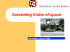### l l f National Geo-spatial Information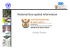### Stata Press presents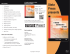### The Analysis of Spatial Association by Use of Distance Statistics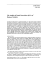### Stata Press presents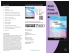### Latvia local level practical example Dviete rural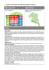### geo saude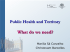### The company operates more than 40 stores in 7 states. 10 SPOT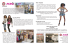### Buoyant Memory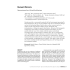### SmartOpenData Leaflet